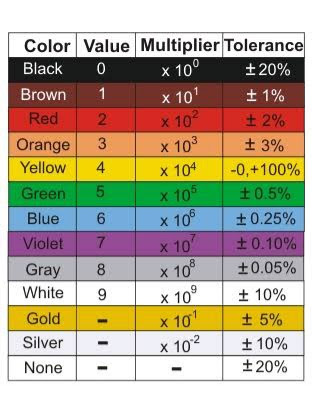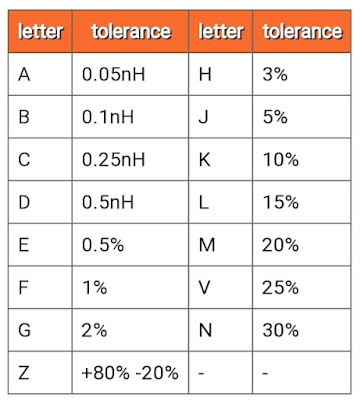## Definition of Inductor

A conducting wire wound in the form of a coil is called an Inductor. When current is passed through it, a magnetic field is formed around the coil and a electromotive force(emf) is generated across two ends of the coil which opposes the change in  flow of current through coil.

Thus the current which flows through inductor is controlled by emf or Voltage that is why it is used for current controlling. Formula for emf is

e= -L dI/dt

L = Inductance of indicator in Henry
I = Current flowing through inductor

## How Inductors Work?

We can understand inductor by a simple hydraulic turbine example in which turbine or water wheel coupled with a heavy fly wheel. Turbine is placed in flowing water and fly wheel is connected with Alternator shaft when water flow then by pressure of water turbine  moves which also moves flywheel. In starting due to inertia fly wheel opposes movement and starts moving slowly but when it reached at its full speed and we stop flow of water then it also opposes stopping of movement and reduces speed slowly thus the fly wheel tries to oppose sudden change in speed. To understand inductor we can assume current flow as water flow, inductance as fly wheel inertia and momentum of flywheel as magnetic flux.

Momentum (magnetic flux) =Inertia (Inductance) × water Flow rate (electric current)

The Inertia of an invisible flywheel (Inductor) that tends to keep the same current flowing can be considered as inductance.

As we increase the weight of fly wheel inertia of fly wheel increases similarly as we increase inductor size inductance of inductor increases.

## Uses of inductor:-

1. It is used to store magnetic energy and transfer of power in the form of magnetic flux with electric isolation.
2. It is used in chokes, filters and attenuators as it passes low frequency and block high frequency.
Because Inductive reactance is directly proportional to frequency which is clear from below
XL = 2πfL

Where f is frequency of current and L is inductance of inductor in Henry.

3. Inductors are also used in tuned circuit for selection of desired frequency in radio receivers.

## Types of Inductor:-

According to core used for wounding conducting wire three types of Inductor  are available
1. Air Core Inductor
2. Iron Core Inductor
3. Ferrite Core Inductor

## Combination of Inductor:-

1. Series Combination:-

Fig-1 and Fig-2 shows inductor connected in series first one connected cumulatively and second one deferentially

Leq = L + L2  + 2M                         (cumulatively connected series Inductor)

Leq = L + L2  - 2M                            (deferentially connected series Inductor)

Fig-3 and Fig-4 shows parallel connected inductors connected with cumulative and differential coupling respectively

Leq  =  (L1   ×   L2-  M 2) / (L +   L2  - 2M)
(cumulatively connected parallel Inductor)

Leq  =  (L1   ×   L2  - M 2 ) / (L +   L+ 2M)
(deferentially connected parallel Inductor)

where M = K× √  (L1   ×   L2
K = coefficient of coupling

## How to find value of Inductor:-Fig1. Inductor colour coding chartFig 2. Inductors tolerance chart

#### For colour coded inductors and numeric coded  inductors value of inductance is find out similar to resistors. First two digit written as it is and third one used as multiplier. Last digit or color code tells about tolerance of inductors.

Green base element is inductor and blue base element is resistor.